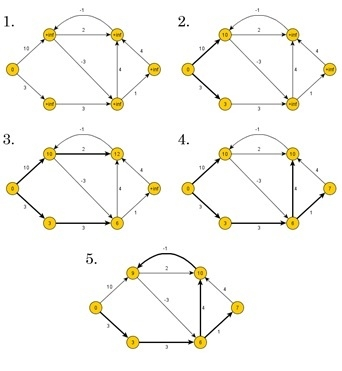# Bellman鈥揊ord Algorithm for Shortest Paths

Bellman-Ford algorithm is used to find minimum distance from the source vertex to any other vertex. The main difference between this algorithm with Dijkstra’s the algorithm is, in Dijkstra’s algorithm we cannot handle the negative weight, but here we can handle it easily.Bellman-Ford algorithm finds the distance in a bottom-up manner. At first, it finds those distances which have only one edge in the path. After that increase the path length to find all possible solutions.

## Input and Output

Input:
The cost matrix of the graph:
0  6  ∞ 7  ∞
∞  0  5 8 -4
∞ -2  0 ∞  ∞
∞  ∞ -3 0  9
2  ∞  7 ∞  0

Output:
Source Vertex: 2
Vert:   0   1   2   3   4
Dist:  -4  -2   0   3  -6
Pred:   4   2  -1   0   1
The graph has no negative edge cycle

## Algorithm

bellmanFord(dist, pred, source)

Input − Distance list, the predecessor list, and the source vertex.
Output − True, when a negative cycle is found.

Begin
iCount := 1
maxEdge := n * (n - 1) / 2     //n is number of vertices

for all vertices v of the graph, do
dist[v] := ∞
pred[v] := 蠒
done

dist[source] := 0
eCount := number of edges present in the graph
create edge list named edgeList

while iCount < n, do
for i := 0 to eCount, do
if dist[edgeList[i].v] > dist[edgeList[i].u] + (cost[u,v] for edge i) dist[edgeList[i].v] > dist[edgeList[i].u] + (cost[u,v] for edge i) pred[edgeList[i].v] := edgeList[i].u
done
done

iCount := iCount + 1
for all vertices i in the graph, do
if dist[edgeList[i].v] > dist[edgeList[i].u] + (cost[u,v] for edge i),
then return true
done

return false
End

## Example

#include<iostream>
#include<iomanip>
#define V 5
#define INF 999
using namespace std;
//Cost matrix of the graph (directed) vertex 5

int costMat[V][V] = {
{0, 6, INF, 7, INF},
{INF, 0, 5, 8, -4},
{INF, -2, 0, INF, INF},
{INF, INF, -3, 0, 9},
{2, INF, 7, INF, 0}
};

typedef struct {
int u, v, cost;
}edge;

int isDiagraph() {

//check the graph is directed graph or not

int i, j;
for(i = 0; i<V; i++) {
for(j = 0; j<V; j++) {
if(costMat[i][j] != costMat[j][i]) {
return 1;      //graph is directed
}
}
}
return 0;//graph is undirected
}

int makeEdgeList(edge *eList) {
//create edgelist from the edges of graph
int count = -1;
if(isDiagraph()) {
for(int i = 0; i<V; i++) {
for(int j = 0; j<V; j++) {
if(costMat[i][j] != 0 && costMat[i][j] != INF) {
count++;         //edge find when graph is directed
eList[count].u = i; eList[count].v = j;
eList[count].cost = costMat[i][j];
}
}
}
}else {
for(int i = 0; i<V; i++) {
for(int j = 0; j<i; j++) {
if(costMat[i][j] != INF) {
count++;         //edge find when graph is undirected
eList[count].u = i; eList[count].v = j;
eList[count].cost = costMat[i][j];
}
}
}
}
return count+1;
}

int bellmanFord(int *dist, int *pred,int src) {
int icount = 1, ecount, max = V*(V-1)/2;
edge edgeList[max];

for(int i = 0; i<V; i++) {
dist[i] = INF;      //initialize with infinity
pred[i] = -1;      //no predecessor found.
}

dist[src] = 0;//for starting vertex, distance is 0

ecount = makeEdgeList(edgeList);       //edgeList formation

while(icount < V) {       //number of iteration is (Vertex - 1)
for(int i = 0; i<ecount; i++) {
if(dist[edgeList[i].v] > dist[edgeList[i].u] + costMat[edgeList[i].u][edgeList[i].v]) {      //relax edge and set predecessor
dist[edgeList[i].v] = dist[edgeList[i].u] + costMat[edgeList[i].u][edgeList[i].v];
pred[edgeList[i].v] = edgeList[i].u;
}
}
icount++;
}

//test for negative cycle
for(int i = 0; i<ecount; i++) {
if(dist[edgeList[i].v] > dist[edgeList[i].u] + costMat[edgeList[i].u][edgeList[i].v]) {
return 1;    //indicates the graph has negative cycle
}
}

return 0;     //no negative cycle
}

void display(int *dist, int *pred) {
cout << "Vert: ";
for(int i = 0; i<V; i++)
cout <<setw(3) << i << " ";
cout << endl;
cout << "Dist: ";

for(int i = 0; i<V; i++)
cout << setw(3) << dist[i] << " ";
cout << endl;
cout << "Pred: ";

for(int i = 0; i<V; i++)
cout << setw(3) << pred[i] << " ";
cout << endl;
}

int main() {
int dist[V], pred[V], source, report;
source = 2;
report = bellmanFord(dist, pred, source);
cout << "Source Vertex: " << source<<endl;
display(dist, pred);

if(report)
cout << "The graph has a negative edge cycle" << endl;
else
cout << "The graph has no negative edge cycle" << endl;
}

## Output

Source Vertex: 2
Vert:   0   1   2   3   4
Dist:  -4  -2   0   3  -6
Pred:   4   2  -1   0   1
The graph has no negative edge cycle